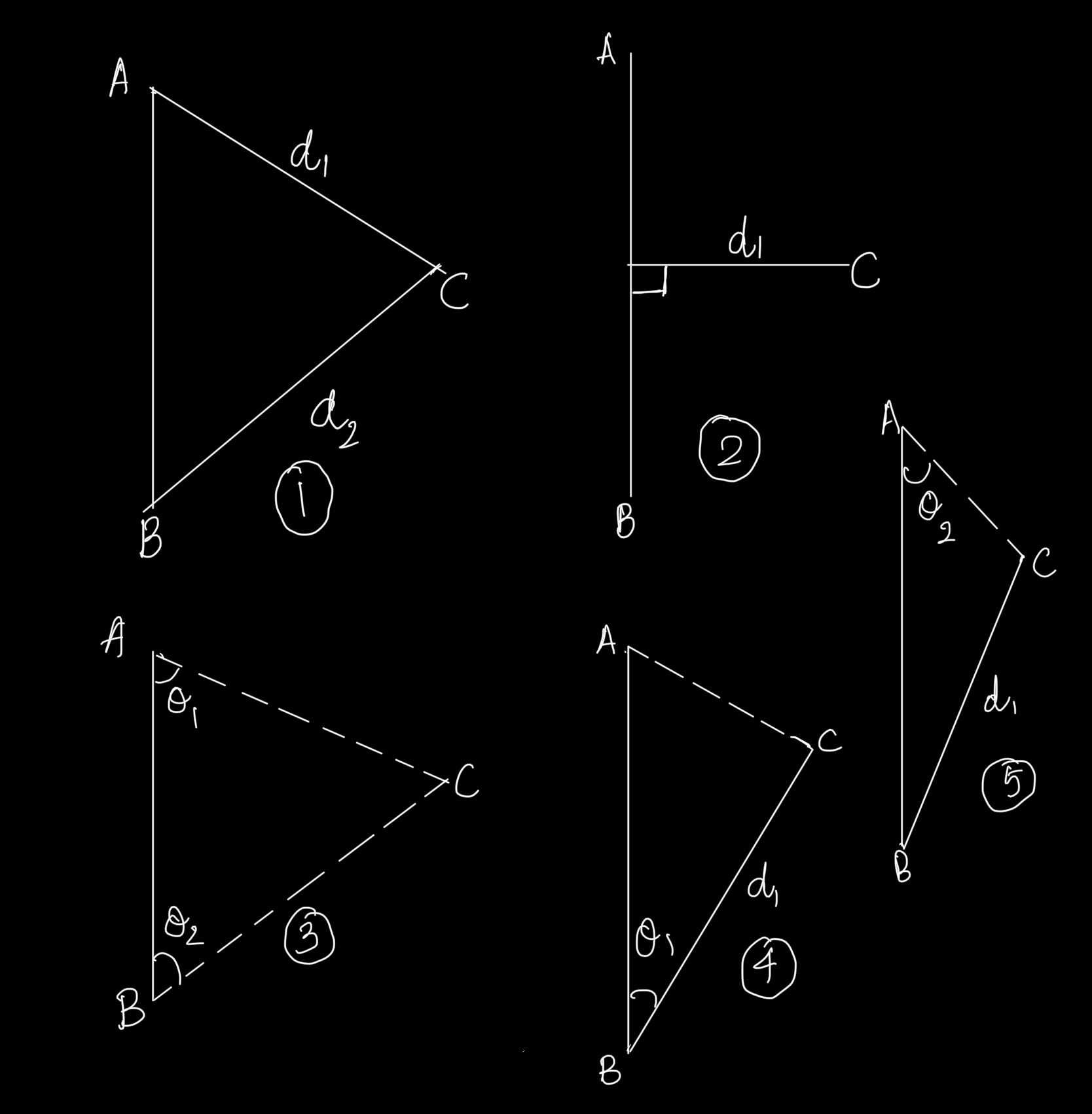##### Question

In: Civil Engineering

# Principles of surveying

Explain in detail, the fundamental principles of surveying in civil engineering.

## Solutions

##### Expert Solution

Surveying is the art of establishing points relative to another known points. For establishing points, there should be convenient and standard principles all over the world. Thus, the fundamental principles are set up.

The first being the working from whole to part concept. For instance, if we have to assess a land given, we can either split into parts by establishing points near to the surveyor and then work to get the whole land mass surveyed, or it can be the other way. If we go by working from part to whole, the error induced will be so high that the setting up of the points  on the periphery becomes really difficult. Whereas if we work from whole to part, the errors will be less and easily identifiable.

Next is the point establishment using at least two measurements from a fixed reference points. Fixed reference point can be a benchmark or a point where we know the details beforehand. Measurement can be linear or angular. As we locate points over circle or any shape, we require at least two measurements for plotting a point. It can be either linear or angular. We can have 5 different ways to plot a point thus on land.As it is seen in the figure, the first part shows the use of two linear measurements where as third part shows the use of two angular measurements. The second part depicts the use of bisection, i.e., angle 90 degrees and linear measurement for establishing a point. The fourth figure shows the use of included angle in establishing point and fifth one shows the use of linear measurement and opposite angle for establishing a point w.r.t a known line/points.

The fundamental principles of surveying include:
1. Working from whole to part

2. Locate a new point by at least two measurements from a fixed reference point